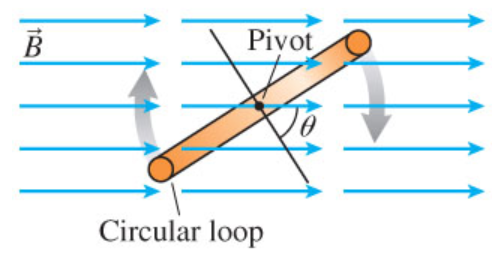# Problem: Figure is an edge-on view of a 16 cm diameter circular loop rotating in a uniform 6.4×10−2 T magnetic fieldA) What is the magnetic flux through the loop when θ is 0∘?B) What is the magnetic flux through the loop when θ is 30∘?C) What is the magnetic flux through the loop when θ is 60∘?D) What is the magnetic flux through the loop when θ is 90∘?

###### FREE Expert Solution

Magnetic flux:

$\overline{){{\mathbf{\varphi }}}_{{\mathbf{B}}}{\mathbf{=}}{\mathbf{B}}{\mathbf{A}}{\mathbf{c}}{\mathbf{o}}{\mathbf{s}}{\mathbf{\theta }}}$, where B is the magnetic field, A is the area, and θ is the angle between B and A.

Area of a circular loop:

$\overline{){\mathbf{A}}{\mathbf{=}}{{\mathbf{\pi r}}}^{{\mathbf{2}}}}$, where r is the radius of the circular loop.

The diameter of the circular loop is 16cm

Therefore, radius, r = 16/2 = 8 cm = 0.08 m

80% (73 ratings)###### Problem Details

Figure is an edge-on view of a 16 cm diameter circular loop rotating in a uniform 6.4×10−2 T magnetic fieldA) What is the magnetic flux through the loop when θ is 0∘?
B) What is the magnetic flux through the loop when θ is 30∘?
C) What is the magnetic flux through the loop when θ is 60∘?
D) What is the magnetic flux through the loop when θ is 90∘?